## About Nathan Bowler

I'm working at the university of Hamburg on infinite matroid theory.

# The infinite matroid intersection conjecture

Today we’ll return to our examination of infinite matroids. So far we saw why they are defined the way they are and what the known examples look like. Then we examined a very flexible way of building infinite matroids from trees of finite matroids and saw how to use that construction as a tool in topological infinite graph theory.

The aim today is to understand the deepest and most important unproved conjecture about infinite matroids, the infinite matroid intersection conjecture. We won’t be looking at progress towards the conjecture today, just approaching the statement from a number of angles and getting a sense of its connections to various very different-looking problems in infinite combinatorics. I hope that by the end of the post you will be convinced, as I am, that it is a deep and compelling problem. Here it is:

Conjecture (Nash-Williams): Let $M$ and $N$ be (possibly infinite) matroids on the same ground set $E$. Then there are a subset $I$ of $E$ and a partition of $E$ into sets $P$ and $Q$ such that $I$ is independent in both $M$ and $N$, $I \cap P$ spans $P$ in $M$ and $I \cap Q$ spans $Q$ in $N$.

Like a TARDIS, at a first glance this statement seems simple and perhaps a little odd, and its deeper significance is hidden. To get a sense of that significance, we must go on a long journey and see how it materialises within apparently widely separated worlds.

Our journey begins with the observation that finding good infinite versions of theorems about finite combinatorial objects is hard. All too often, the obvious generalisation is either straightforwardly false or else is a simple consequence of the finite version of the theorem, and as such has no new content.

An example of the latter phenomenon is Menger’s Theorem. If $G$ is a graph and $A$ and $B$ are sets then an $A$-$B$ separator in $G$ is defined to be a set $S$ of vertices of $G$ such that there is no path from $A$ to $B$ in $G – S$. Menger’s theorem states that if $G$ is finite then the minimal size of an $A$-$B$ separator in $G$ is the same as the maximal size of a set of disjoint paths from $A$ to $B$ in $G$.

The obvious way to generalise this statement to infinite graphs would be to simply replace the word ‘size’ with the word ‘cardinality’ in both places where it appears. However, the statement obtained in this way has no more content than the finite version of the theorem. We can see this by considering an $A$-$B$ separator $S$ of minimal cardinality.

If $S$ is infinite, then any set of fewer than $|S|$ paths from $A$ to $B$ uses fewer than $|S|$ vertices, and so cannot be maximal. So in that case the statement is clear, and we can suppose instead that $|S|$ is some natural number $n$. Now for each $m \leq n$ we can easily build a finite subgraph $G_m$ of $G$ in which any $A$-$B$ separator has size at least $m$: we may take $G_0$ to be empty, and build $G_{m+1}$ from $G_m$ by adding a path $P_X$ of $G$ from $A$ to $B$ avoiding each set $X$ of $m$ vertices of $G_m$. Then by Menger’s theorem $G_n$ already contains $n$ disjoint paths from $A$ to $B$.

It was Paul Erdős who saw how to get a much deeper infinite generalisation by first reformulating Menger’s theorem as a structural statement. Suppose that we consider an $A$-$B$ separator $S$ of minimal size and a set $\cal P$ of disjoint paths from $A$ to $B$ of maximal size. Then each path in $\cal P$ contains at least one vertex in $S$, and these vertices must all be distinct since the paths are disjoint. But by Menger’s theorem there can only be as may paths in $\cal P$ as there are vertices in $S$. So $S$ must consist of one vertex on each path in $\cal P$.

So it follows from Menger’s theorem that in a finite graph $G$ we can always find a set $\cal P$ of disjoint $A$-$B$ paths together with an $A$-$B$ separator $S$ consisting of one vertex from each path in $\cal P$. On the other hand, this structural statement also implies Menger’s theorem. After all, if ${\cal P}’$ is a set of disjoint paths from $A$ to $B$ of maximal size and $S’$ is an $A$-$B$ separator of minimal size then $|S’| \leq |S| = |{\cal P}| \leq |{\cal P}’|$. But also $|{\cal P}’| \leq |S’|$ since each path in ${\cal P}’$ must contain a different point of $S’$. So $|{\cal P}’| = |S’|$, as desired.

Erdős’ generalisation of Menger’s theorem is therefore the following structural statement:

Theorem (Aharoni and Berger): Let $G$ be a (possibly infinite) graph and let $A$ and $B$ be sets. Then there is a set ${\cal P}$ of disjoint $A$-$B$ paths together with an $A$-$B$ separator $S$ consisting of one vertex from each path in ${\cal P}$.

This statement contains some serious content about the structure of infinite graphs, and it remained open for almost half a century before finally being proved by Aharoni and Berger in 2009 [AB09]. Their proof remains one of the deepest ever given in infinite combinatorics.

Another example of the difficulties of generalisation from finite to infinite objects is given by the tree packing and covering theorems. The tree covering theorem states that a connected graph $G$ is a union of $k$ spanning trees if and only if for any set $X$ of vertices of $G$ the induced subgraph $G[X]$ has at most $k(|X| – 1)$ edges, and the tree packing theorem states that a connected graph $G$ includes $k$ edge-disjoint spanning trees if and only if for any partition $P$ of the vertex set of $G$, the quotient graph $G/P$ has at least $k(|P|-1)$ edges. Here $G/P$ is the graph whose vertices are the partition classes and whose edges are those of $G$ which go between partition classes, with endpoints the partition classes which they join.

Once more, the obvious generalisation of the tree covering theorem to infinite graphs has no more content than the finite version of the theorem; it can be proved from it by a straightforward compactness argument. On the other hand the obvious generalisation of the tree packing theorem to infinite graphs is false; there is a counterexample due to Aharoni and Thomassen [AT89]. And once more, to find the correct infinite version of the theorems we must begin by finding a structural version in the finite case. Indeed, it turns out that the tree packing and covering theorems have a unifying structural generalisation:

Theorem ([D17, Theorem 2.4.4]): Let $G$ be a connected finite graph and $k$ a natural number. Then there is a partition $P$ of the vertex set of $G$ such that $G/P$ is a union of $k$ spanning trees and $G[X]$ is connected and has $k$ edge-disjoint spanning trees for each partition class $X$ of $P$.

This tree packing/covering theorem implies both the tree packing theorem and the tree covering theorem. For tree packing, the necessity of the condition is clear, so it suffices to prove sufficiency. We can do this by applying the condition to the partition $P$ given by the tree packing/covering theorem. This gives that $G/P$ has at least $k(|P|-1)$ edges. Since it is a union of $k$ spanning trees, those trees must be edge disjoint. Combining these with the edge-disjoint spanning trees in each $G[X]$ gives $k$ edge-disjoint spanning trees in $G$. The derivation of the tree covering theorem from the packing/covering theorem is similar.

This gives us a nontrivial common generalisation of the tree packing and covering theorems to infinite graphs: we can simply omit the word ‘finite’ from the tree packing/covering theorem. The proof of this generalisation, though much simpler than that for the infinite version of Menger’s theorem, goes beyond the scope of this post.

We have now seen two examples where, to find the correct infinite generalisation of a theorem about finite graphs, it was necessary to first reformulate the finite theorem as a structural result. The same is true for theorems about finite matroids, but in this case something remarkable happens. The infinite structural statement you get is usually just the infinite matroid intersection conjecture!

This is not too surprising for the matroid intersection theorem, since Nash-Williams formulated the intersection conjecture to be an infinite structural generalisation of that statement. Recall that the matroid intersection theorem states that the largest size of a common independent set of two matroids $M$ and $N$ on the same ground set $E$ is the same as the minimum value over all partitions of $E$ into sets $P$ and $Q$ of $r_M(P) + r_N(Q)$. The inequality one way around is clear, since if $I$ is independent in both $M$ and $N$ and $\{P, Q\}$ is a partition of $E$ then $|I| = |I \cap P| + |I \cap Q| \leq r_M(P) + r_N(Q)$. For this inequality to be an equality, we must have that $I \cap P$ spans $P$ in $M$ and $I \cap Q$ spans $Q$ in $N$, just as in the conjecture.

There are some less expected cases. Let’s consider Tutte’s linking theorem, the closest matroidal analogue of Menger’s theorem. Let $M$ be a finite matroid with ground set $E$, and let $A$ and $B$ be disjoint subsets of $E$. Let $E’ := E \setminus (A \cup B)$. Then the connectivity $\lambda_M(A, B)$ from $A$ to $B$ in $M$ is defined to be the minimal value of $\kappa_M(A \cup P)$ over all bipartitions of $E’$ into sets $P$ and $Q$. Here $\kappa_M$ is the connectivity function of $M$, given by $\kappa_M(X) := r_M(X) + r_M(E \setminus X) – r(M)$. The linking theorem states that the maximum value of $\kappa_N(A)$ over all minors $N$ of $M$ with ground set $A \cup B$ is $\lambda_M(A,B)$.

It turns out that there is a structural analogue of this statement. Each such minor $N$ must have the form $M/I\backslash J$, where $I$ and $J$ form a partition of $E’$. By moving loops of $M/I$ into $J$ if necessary, we may suppose that $I$ is independent. We may now calculate as follows:

$\kappa_{M/I \setminus J}(A) = (r(A \cup I) – |I|) + (r(B \cup I) – |I|) – (r(M) – |I|) \\= (r(A \cup I) – |Q \cap I|) + (r(B \cup I) – |P \cap I|) – r(M) \\ \leq r(A \cup (I \cap P)) + r(B \cup (I \cap Q)) – r(M) \\ \leq r(A \cup P) + r(B \cup Q) – r(M) \\ = \kappa_M(A \cup P)$

So equality of the left and right sides is equivalent to the statement that each inequality in the above calculation is an equality, giving the following four conditions:

1. $I \cap P$ spans $P$ in $M/A$
2. $I \cap Q$ spans $Q$ in $M/B$
3. $I \cap P$ is independent in $M/(B \cup (I \cap Q))$
4. $I \cap Q$ is independent in $M/(A \cup (I \cap P))$

The outlines of our TARDIS are beginning to materialise. Indeed, consider a minimal set $I$ satisfying these conditions. By minimality, $I \cap P$ will be independent in $M/A$ and $I \cap Q$ will be independent in $M/B$. Thus $I$ itself will be independent in both matroids. To put it another way, $I$, $P$ and $Q$ will witness that $M/A \backslash B$ and $M \backslash A/B$ satisfy the matroid intersection conjecture.

Thus the infinite generalisation of Tutte’s linking theorem is the statement that, for any (possibly infinite) matroid $M$ and any disjoint sets $A$ and $B$ of elements of $M$, the matroids $M/A\backslash B$ and $M \backslash A/B$ satisfy the infinite matroid intersection conjecture. Given this connection, it should not be too surprising that Aharoni and Berger’s infinite generalisation of Menger’s theorem follows from the infinite matroid intersection conjecture. Precise details of the derivation can be found in [ACF18].

What about the tree packing and covering theorems? Their matroidal analogues are the base packing and covering theorems, which in their full generality apply to a list $M_1, M_2, \ldots M_k$ of finite matroids on a common ground set $E$. A base packing for such a list is a collection of disjoint bases, one from each $M_i$. A base covering for such a list is a collection of bases, one from each $M_i$, whose union is the whole of $E$. The base packing theorem states that there is a base packing precisely when for any subset $Q$ of $E$ we have $\sum_{i = 1}^k r(M_i.Q) \leq |Q|$, and the base covering theorem states that there is a base covering precisely when for any subset $P$ of $E$ we have $\sum_{i = 1}^k r(M_i | P) \geq |P|$.

Once more we can combine these statements into a unified structural statement, the base packing/covering theorem, which states that given such a list of finite matroids on $E$ we can find a bipartition of $E$ into sets $P$ and $Q$ such that the matroids $M_1 | P, \ldots M_k | P$ have a packing and the matroids $M_1.Q, \ldots M_k.Q$ have a covering. The derivations of the base packing and covering theorems from this statement are analogous to the derivation of the tree packing theorem from the tree packing/covering theorem above. So the infinite version of the base packing and covering theorems is given by the same statement applied to a family of infinite matroids. We shall call this the base packing/covering conjecture.

Let’s consider the special case $k = 2$ in more detail. The existence of a packing for $M_1 | P$ and $M_2 | P$ is equivalent to the existence of a subset $I_P$ of $P$ such that $I_P$ spans $P$ in $M_1$ and $P \setminus I_P$ spans $P$ in $M_2$. Similarly the existence of a covering for $M_1.Q$ and $M_2.Q$ is equivalent to the existence of a subset $I_Q$ of $Q$ such that $I_Q$ is independent in $M_1/P$ and $Q \setminus I_Q$ is independent in $M_2/P$. Since a set is independent in a matroid precisely when its complement is spanning in the dual matroid, we can rephrase these conditions as follows:

1. $I_P$ spans $P$ in $M_1$
2. $I_Q$ spans $Q$ in $M_2^*$
3. $I_P$ is independent in $M_2^*/Q$
4. $I_Q$ is independent in $M_1/P$

Once again, as if from nowhere, the TARDIS appears. If we choose $I_P$ and $I_Q$ minimal subject to conditions (i) and (ii) then they will still satisfy conditions (iii) and (iv), which will guarantee that $I:=I_P \cup I_Q$ is independent in both $M_1$ and $M_2^*$, meaning that $I$, $P$ and $Q$ witness that $M_1$ and $M_2^*$ satisfy the infinite matroid intersection conjecture.

The TARDIS not only appears in unexpected places, it is also bigger on the inside than it seems. For example, the remarks in the last couple of paragraphs only apply to pairs of matroids, that is, to lists of length 2. But in fact it is possible to derive the full base packing/covering conjecture from the special case of pairs, and hence from the infinite matroid intersection conjecture. We will see the reasons for this when we look at the structure of the conjecture more closely in the next post in the series. For now we just note the consequence that the tree packing/covering theorem mentioned earlier also follows from the infinite matroid intersection conjecture.

We have seen how the infinite matroid intersection conjecture arises naturally as the infinite structural analogue of the matroid intersection theorem, the linking theorem, and the base packing and covering theorems. The same also holds for the matroid union theorem, which we do not have space to discuss here [BC15]. Thus the process of finding an infinite generalisation of all these statements reveals their unified structural heart. In the next post we will examine that structural heart more closely, looking at just what sort of structure the conjecture gives us, and we will survey the special cases for which the conjecture is already known.

Bibliography:

[AB09] R. Aharoni and E. Berger, Menger’s Theorem for Infinite Graphs, Inventiones mathematicae 176(1):1–62 (2009).

[ACF18] E. Aigner-Horev, J. Carmesin and J.-O. Fröhlich, On the Intersection of Infinite Matroids, Discrete Mathematics 341(6):1582-1596 (2018).

[AT89] R. Aharoni and C. Thomassen, Infinite, highly connected digraphs with no two arc-disjoint spanning trees. J. Graph Theory, 13:71–74 (1989).

[BC15] N. Bowler and J. Carmesin, Matroid Intersection, Base Packing and Base Covering for Infinite Matroids, Combinatorica 35(2):153-180 (2015).

[D17] R. Diestel, Graph Theory, 5th edition, Springer-Verlag (2017).

# The matroid union is back!

It has been almost a year, but now the Matroid Union is back. Just as before, we’re planning to have a new post every 2 weeks on Monday. Over the next month Laura Anderson will be writing about a new application of oriented matroids in mathematical psychology, and I will introduce the deepest problem in the theory of infinite matroids, the infinite matroid intersection conjecture.

The team of core contributors has changed a little; we’re sorry to say goodbye to Stefan van Zwam and Irene Pivotto, who put a lot of energy into making this blog what it is. But we have some fresh new contributors: Laura Anderson and Nick Brettell. You can get some idea of what topics we are each hoping to cover here. A variety of other topics will be covered by guest posts. If you have any ideas for topics which you would like to see on the blog, or even which you would like to write about yourself, then please get in touch.

# Building matroids from infinite graphs

Today we’ll be looking at infinite matroids again. We started this series by examining the question of how infinite matroids could be defined. With a suitable definition in hand, we took a quick tour through the zoo of known examples. Then we took a closer look at one very flexible way to build infinite matroids: by sticking together infinite trees of matroids.

In that construction we used 2-sums to stick the matroids together. Suppose that we have a finite collection of matroids arranged in a tree, so that their ground sets overlap (always in single edges) if and only if they are adjacent in the tree. Then because 2-sums are associative we have an unambiguously defined 2-sum of that collection. In the previous post in this series, we saw that this construction also works if we allow the tree to be infinite, but that we have to specify a little extra information the set $\Psi$ of ends of the tree which the circuits are allowed to use.

The same trick can be used for other kinds of associative sum of finite matroids. In this post we’ll see how it works for sums of representable matroids, and why that is useful for understanding the topological spaces associated to infinite graphs.

To see how the addition of representable matroids works, we need to look at them from a slightly unusual angle. Let’s say that we have a matroid $M$ on a ground set $E$, and suppose that we have vectors $\{v_e | e \in E\}$ in some vector space over a field $k$, giving us a representation of $M$ over $k$. Then we can capture all the essential information about this representation by forgetting the details of the vector space and focusing just on the linear relationships amongst the vectors. More formally, we say that an element $\lambda$ of the space $k^E$ is a linear dependence of the $v_e$ if $\sum_{e \in E} \lambda(e)v_e = 0$. Then the linear dependences form a subspace $V$ of $k^E$, and this subspace is enough to recover the matroid $M$; the circuits of $M$ are precisely the minimal nonempty supports of vectors in $V$. For those who prefer to think of representations in terms of matrices rather than families of vectors, the subspace we’re working with is just the orthogonal complement of the row space of the matrix.

So we can encode representations of matroids on $E$ over $k$ as subspaces of $k^E$. This way of seeing representations fits well with matroid duality, in that if $V \subseteq k^E$ represents a matroid $M$ on $E$ then the orthogonal complement $V^{\bot}$ represents the dual matroid $M^*$. If we define $M(V)$ to be the matroid whose circuits are the minimal nonempty supports of elements of $V$, then we can express this as $M(V^{\bot}) = (M(V))^*$.

The advantage of this perspective is that there is a natural way to glue together such subspaces, which we can use to build a self-dual gluing operation for represented matroids. Suppose that we have two sets $E_1$ and $E_2$ and subspaces $V_1$ and $V_2$ of $k^{E_1}$ and $k^{E_2}$, respectively. We now want to glue these together to give a subspace $V_1 \oplus V_2$ of $E_1 \triangle E_2$. As with the 2-sum, we throw away the gluing edges’ in the overlap $E_1 \cap E_2$. The idea is to take pairs of vectors which match on the gluing edges, patch them together and throw away the part supported on the gluing edges. More precisely, we set $V_1 \oplus V_2 := \{v \mathord{\upharpoonright}_{E_1 \triangle E_2} | v \in k^{E_1 \cup E_2}, v \mathord{\upharpoonright}_{E_i} \in V_i\}$.

Like the 2-sum, this definition is self-dual in the sense that $(M(V_1 \oplus V_2))^* = M(V_1^{\bot} \oplus V_2^{\bot})$. It is also associative, in that if $V_1$, $V_2$ and $V_3$ are subspaces of $k^{E_1}$, $k^{E_2}$ and $k^{E_3}$ respectively and the sets $E_1$ and $E_3$ are disjoint then $(V_1 \oplus V_2) \oplus V_3 = V_1 \oplus (V_2 \oplus V_3)$. So if we have a finite collection of such representations on ground sets $E_t$ arranged in a finite tree, such that the ground sets only overlap if they are adjacent in the tree, then we have an unambiguous sum of all these subspaces.

Just as for 2-sums, we can also glue together infinite trees of represented matroids in this way, as long as we are careful to specify which ends of the tree the circuits are allowed to use. Formally, we do this as follows. Suppose that we have a tree $T$, a family of sets $E_t$ indexed by the nodes of $T$, such that $E_s \cap E_t$ is only nonempty if $s = t$ or $s$ and $t$ are adjacent in $T$, a family of subspaces $V_t \subseteq k^{E_t}$ and a Borel subset $\Psi$ of the set $\Omega(T)$ of ends of $T$. Then we can build a subspace of $k^{\bigtriangleup_{t}E_t}$ by setting

$\bigoplus^{\Psi}_t V_t := \{v \mathord{\upharpoonright}_{\bigtriangleup_t E_t} | v \in k^{\bigcup_t E_t}, v \mathord{\upharpoonright}_{E_t} \in V_t \text{ and } \Omega(T) \cap \overline{\{t | v \upharpoonright_{E_t} = 0\}} \subseteq \Psi\}$

and $M(\bigoplus^{\Psi}_t V_t)$ will be an infinite matroid.

What are these infinite sums good for? Well, if we have a representable matroid and we have a $k$-separation of that matroid then we can split it up as a sum of two matroids in this way such that there are fewer than $k$ gluing edges. We can use this to break problems about bigger matroids down into problems about smaller matroids. Similarly, if we have a nested collection of finite separations in an infinite matroid, cutting the ground set up into a tree of finite parts, then we can cut the matroid up into a sum of finite matroids and analyse its properties in terms of their properties. This kind of chopping up and reconstruction can also be helpful to show that the infinite object is a matroid in the first place.

Let’s see how that might work for a more concrete problem. Suppose that we have a locally finite graph $G$. Then we can build a topological space $|G|$ from it by formally adding its ends as new points at infinity (see for example [D10]). These spaces and their subspaces are key elements of topological infinite graph theory, which was where this series of posts started.

At first, it was hoped that these subspaces would have the nice property that if they are connected then they are path connected. But Agelos Georgakopoulos eventually found a counterexample to this claim [G07]. However, the set of ends used by the counterexample he constructed was topologically horrible, so we might still hope that if we have a connected subspace $X$ of $|G|$ such that the set $\Psi$ of ends contained in $X$ is topologically nice, then $X$ will be path-connected. Well, if we take topologically nice’ to mean Borel’, then the ideas above let us show that this is true.

We can do this by considering the matroid whose circuits are the edge sets of those topological circles in $|G|$ which only go through ends in $\Psi$. More precisely, we need to show that this really does give the circuit set of a matroid $M(G, \Psi)$. If we can do that, then we can argue as follows:

Let $P$ be the set of edges which, together with both endpoints, are completely included in $X$. Let $u$ and $v$ be vertices in $X$. Build a new graph $G + e$ with an extra edge $e$ joining $u$ to $v$. Then since $X$ is connected, there can be no cocircuit of $M(G + e, \Psi)$ which contains $e$ and is disjoint from $P$ (such a cocircuit would induce a topological separation of $X$ with $u$ and $v$ on opposite sides). So $e$ is not a coloop in the restriction of $M(G + e, \Psi)$ to $P \cup \{e\}$. Hence there is a circuit through $e$ in that matroid, and removing $e$ from the corresponding topological circle gives an arc from $u$ to $v$ through $X$ in $|G|$. So any two vertices are in the same path-component of $X$. Similar tricks show the same for ends and interior points of edges.

What this argument shows is that the connection between connectivity and path-connectivity is encoded in the statement that $M(G, \Psi)$ is a matroid. To prove that statement, we can build $M(G, \Psi)$ as the sum of an infinite tree of graphic matroids in the sense described above. First of all, since $G$ is locally finite, we can cut it up into an infinite tree of finite connected parts using disjoint finite separators. Then we define the torso corresponding to a part to consist of that part together with new edges forming complete graphs on each of the separators. This gives us an infinite tree of finite graphs, and the ends of the tree correspond precisely to the ends of $G$. Now we take the graphic matroids corresponding to those graphs, take the standard binary representations of those matroids, and glue them together along this tree, taking the ends in $\Psi$ to be allowed for circuits. And presto! We have build the matroid $M(G, \Psi)$.

The details of this argument are explained in [BC15].

I can’t resist mentioning that the matroids we’ve just built in a bottom-up way also have a top-down characterisation. Consider the class of matroids whose ground set is the set of edges of $G$, and in which every circuit is a topological circuit of $G$ and every cocircuit is a bond of $G$. Let’s call such matroids $G$-matroids.

For some graphs $G$, we can find $G$-matroids which are not of the form $M(G, \Psi)$. For example, in the following graph $Q$ we can define an equivalence relation on the (edge sets of) double rays by saying that two double rays are equivalent if they have finite symmetric difference. Then the set of finite circuits together with any collection of double rays closed under this equivalence relation gives the set of circuits of an infinite matroid.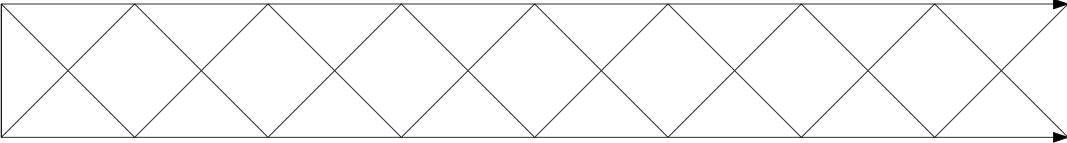The matroids I just described are a bit pathological, and they hover on the boundary between being binary and non-binary. None of them has a $U_{2,4}$-minor. They also still have the familiar property that any symmetric difference of two circuits is a disjoint union of circuits. But symmetric differences of three circuits might not be disjoint unions of circuits!

For example, there is such a matroid in which the first three sets depicted below are circuits, but the fourth, their symmetric difference, is not.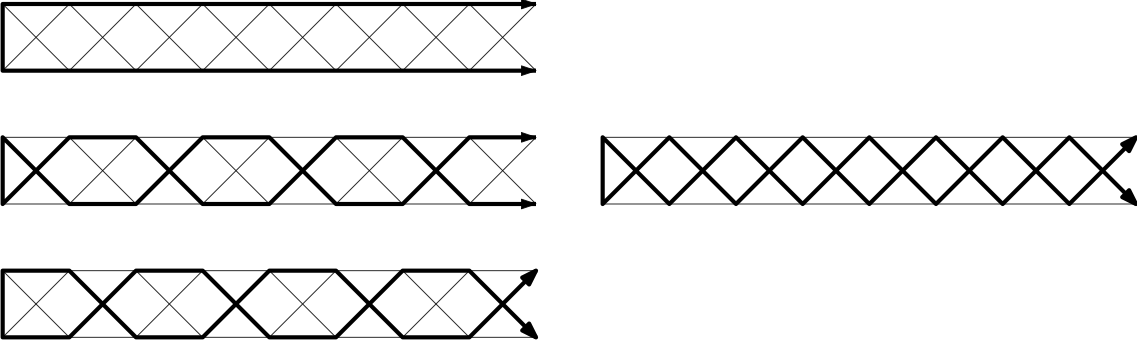The problem is that these matroids are wild. This means that there are circuits and cocircuits which intersect in infinitely many elements. We only have a good theory of representability for tame matroids, those in which every intersection of a circuit with a cocircuit is finite. I hope to discuss this in more detail in a future post.

If we only consider tame $G$-matroids, then this proliferation of pathological matroids disappears. For the graph $Q$, for example, there are only 2 tame $Q$-matroids, namely the finite cycle matroid and the topological cycle matroid. Remarkably, for any locally finite graph $G$ it turns out that the tame $G$-matroids are precisely the matroids of the form $M(G, \Psi)$. So our top-down and bottom up characterisations meet, and any matroid associated to a graph can be built up from finite parts in a way which mirrors the structure of that graph. The reasons for this correspondence go far beyond the scope of this post, but they can for example be found in [BC16].

Now that we’ve seen the standard ways to build infinite matroids and their relationship to infinite graphs, in the next post we’ll examine the most important open problem about them: the Infinite Matroid Intersection Conjecture.

[BC15] N. Bowler and J. Carmesin, Infinite Matroids and Determinacy of Games, preprint here.
[BC16] N. Bowler and J. Carmesin, The ubiquity of Psi-matroids, preprint here.
[D10] R. Diestel, Locally finite graphs with ends: a topological approach I–III, Discrete Math 311–312 (2010–11).
[G07] A. Georgakopoulos. Connected but not path-connected subspaces of infinite graphs, Combinatorica, 27(6) 683–698 (2007).

# Infinite trees of matroids

Welcome back to this series of posts about infinite matroids. Up to this point we’ve seen what infinite matroids are, and we’ve started to understand the notion a little by looking at a variety of examples. Next we will look at how to stick together infinite matroids to build bigger ones. We’ll see that it is possible to stick infinitely many matroids together at once, and this will give us a flexible way to build new infinite matroids by sticking together infinitely many finite matroids.

Let’s start with a familiar way to stick matroids together: via 2-sums. Suppose that we have matroids $M_1$ and $M_2$ with ground sets $E_1$ and $E_2$, and suppose that these ground sets meet in only a single edge $e$. For the sake of simplicity, we’ll assume that $e$ isn’t a loop or a coloop in either matroid. Now we can build a new matroid $M_1 \oplus_2 M_2$ on the ground set $E_1 \triangle E_2$, by taking the circuits to be sets of the following 3 kinds:

• circuits of $M_1$ not containing $e$
• circuits of $M_2$ not containing $e$
• sets of the form $C_1 \cup C_2 -e$, where $C_1$ and $C_2$ are circuits of $M_1$ and $M_2$ respectively, both containing $e$.

These three kinds of circuit are illustrated below: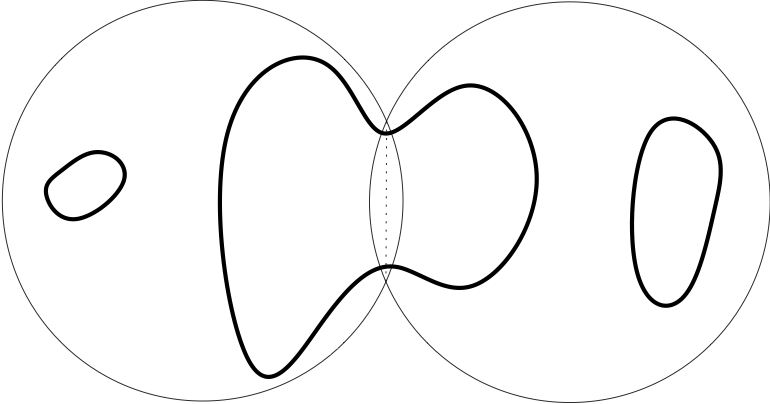This operation is associative, in the sense we might expect: suppose we have 3 matroids $M_1$, $M_2$ and $M_3$ on ground sets $E_1$, $E_2$ and $E_3$. Suppose that $E_1 \cap E_2$ and $E_2 \cap E_3$ each contain just a single edge, and that $E_1 \cap E_3$ is empty. Then the two matroids $M_1 \oplus_2 (M_2 \oplus_2 M_3)$ and $(M_1 \oplus_2 M_2) \oplus_2 M_3$ are equal. This is easy to see by considering the six kinds of circuits which can arise in the sum, as shown here: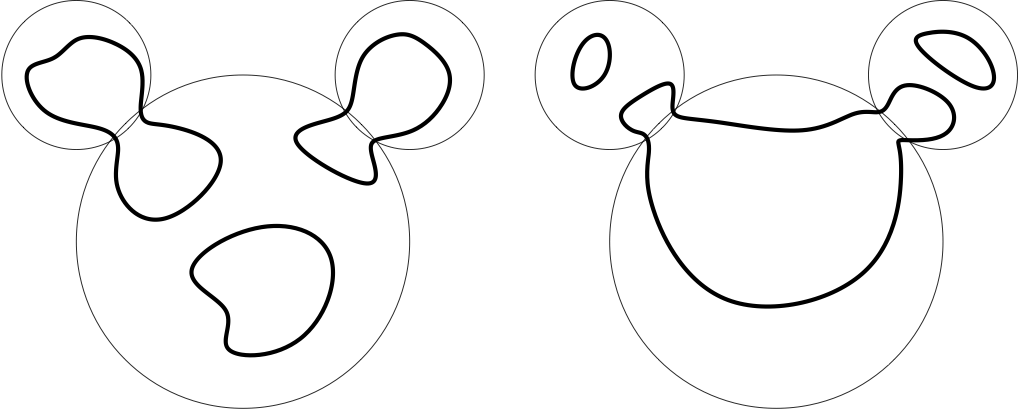Usually associativity means that it doesn’t matter how we bracket a list of things that we want to add together, but in the case of 2-sums the geometry is a little different. The kind of configuration we might want to stick together with 2-sums in general is not a list of matroids, but rather a tree of matroids. More precisely, suppose we have a finite tree $T$, and that for each node $t$ of $T$ we have a matroid $M_t$ with ground set $E_t$. Suppose further that for distinct nodes $t$ and $t’$ of $T$ the intersection $E_t \cap E_{t’}$ either consists of a single edge $e_{tt’}$, if $t$ and $t’$ are neighbours in $T$, or else is empty. Let’s call a structure like this a finite tree of matroids. Then there is an unambiguously defined 2-sum of our tree of matroids, whose ground set is the union of all the sets $E_t$ but without the gluing edges’ $e_{tt’}$. A typical circuit in such a 2-sum of a tree is shown here: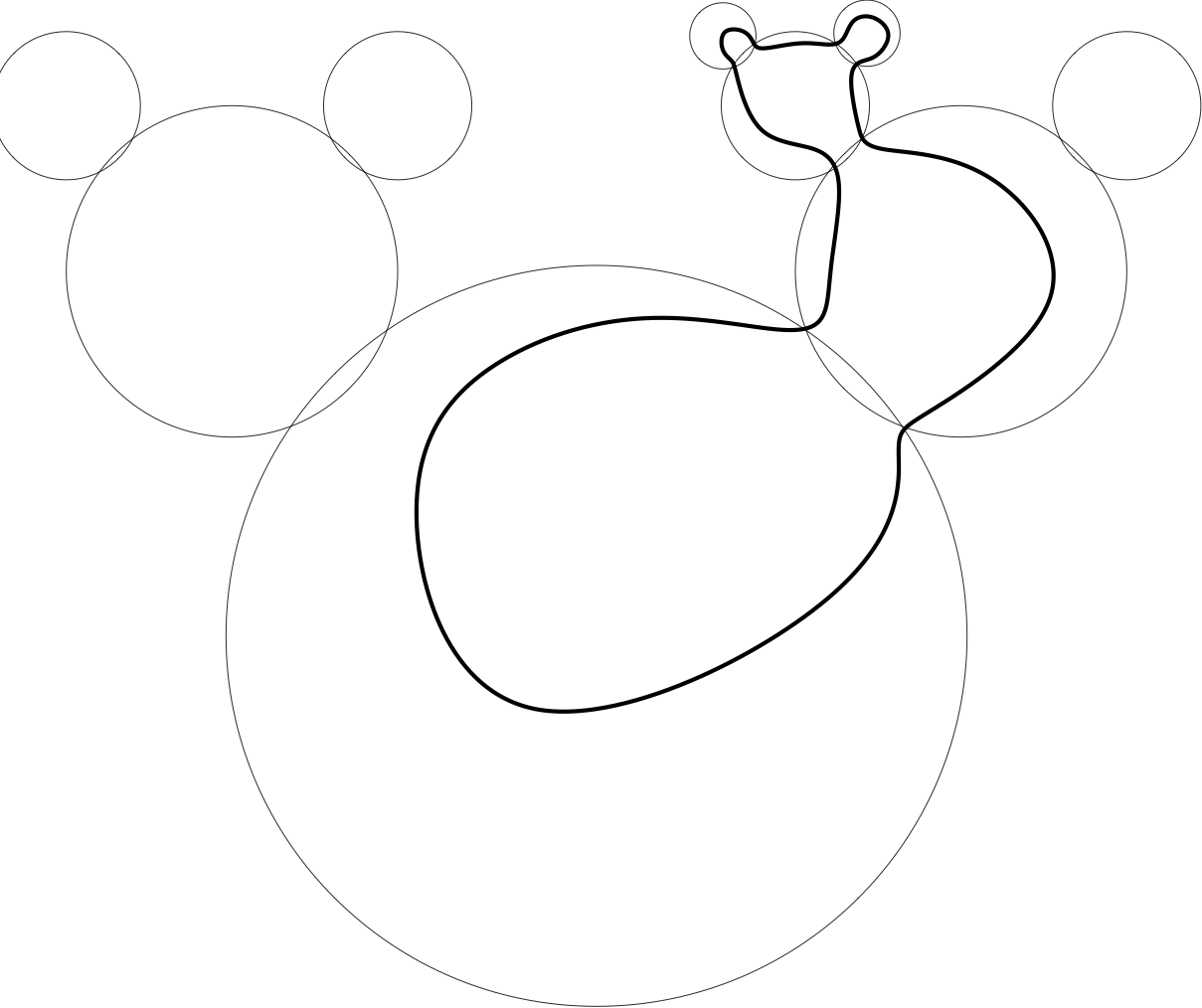More precisely, each circuit of the 2-sum is supported on some subtree $S$ of $T$. It is determined by a choice, for each node $t$ of $S$, of a circuit $C_t$ of $M_t$. This circuit $C_t$ should contain precisely those gluing edges $e_{tt’}$ of $M_t$ such that $t’$ is also in $S$. Given such a subtree $S$ and such a family of circuits $C_t$, we get a circuit of the 2-sum whose edges are the non-gluing edges of the circuits $C_t$.

All of this works just as well for sticking together infinite matroids as for finite ones. But the real power of this technique for building new infinite matroids comes when we consider 2-sums of infinitely many matroids at once.

So what happens if we try to stick together an infinite tree of matroids in the way outlined above? To understand the kinds of difficulty which can arise, let’s first of all consider the simplest possible infinite tree: an infinite ray. We certainly want to allow circuits of the following kind: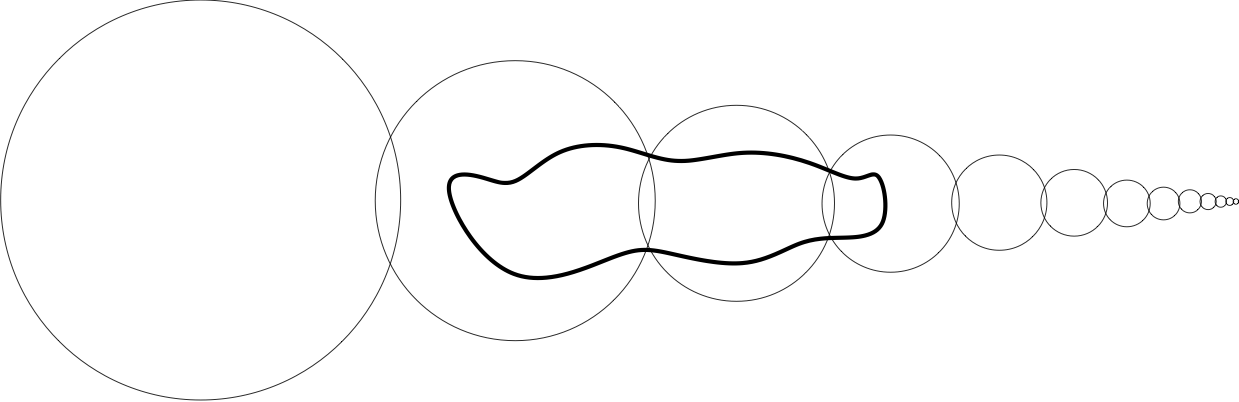But what about ones which go all the way to the end of the ray: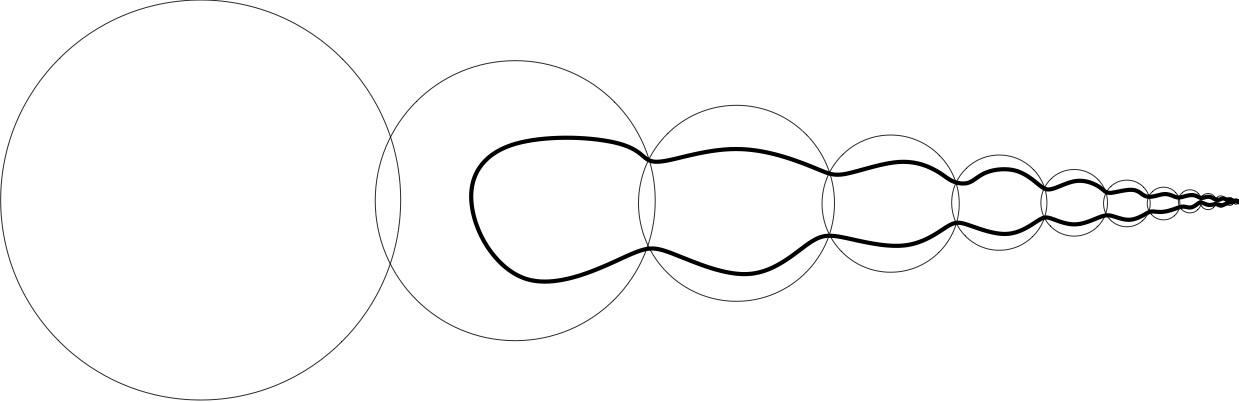Should we allow them?

It turns out that if we allow them, then we get an infinite matroid. But we also get a different infinite matroid by banning them: by only allowing those circuits whose underlying tree $S$ is finite. So we have two different options for how to build a matroid by sticking together an infinite ray of matroids. We say that the circuits are allowed to use the end of the ray in the first of these matroids, but not in the second.

We could just decide to fix one of these two as the `correct’ 2-sum of the ray of matroids, but it turns out that that would be a bad idea. To see why, we should consider the interaction of 2-sums with duality. If we take the dual of the 2-sum of 2 matroids, then it turns out to be the same as the 2-sum of their duals. It follows that the same is true for finite trees of matroids: the dual of the 2-sum of a tree of matroids is the 2-sum of the duals of those matroids. This is certainly a property which we would like to keep for infinite 2-sums.

Now suppose that we have a ray of matroids, and we take the variant of the 2-sum where the circuits are not allowed to use the end, then take the dual of that 2-sum. What we get is the 2-sum of the duals of the matroids, but this time in the version where the circuits are allowed to use the end. So these two different constructions of the 2-sum of a ray are dual to each other, and we shouldn’t privilege either of them.

Formally, we take the decision about whether the circuits should be allowed to use the end of the ray to be part of the data used to specify the sum, in addition to the choices of the matroids lying along the ray. More generally, suppose that we have an infinite tree of matroids. Then in order to specify a 2-sum for this tree of matroids, we must decide, for each end of the tree, whether the circuits are allowed to use that end. Here the ends of the tree can be identified with the rays from a fixed root.

More precisely, suppose that we have a (possibly infinite) tree $T$, and that for each node $t$ of $T$ we have a matroid $M_t$ with ground set $E_t$. Suppose further that for distinct nodes $t$ and $t’$ of $T$ the intersection $E_t \cap E_t’$ either consists of a single edge $e_{tt’}$, if $t$ and $t’$ are neighbours in $T$, or else is empty. Suppose further that we have a subset $\Psi$ of the set of ends of $T$, which we intend to be the set of ends which we will allow circuits to use. Then we might hope to define the 2-sum of this tree of matroids as follows:

Each circuit of the 2-sum will be supported on some subtree $S$ of $T$, such that all rays in $S$ go to ends in $\Psi$. The circuit is determined by a choice, for each node $t$ of $S$, of a circuit $C_t$ of $M_t$. This circuit $C_t$ should contain precisely those gluing edges $e_tt’$ of $M_t$ such that $t’$ is also in $S$. Given such a subtree $S$ and such a family of circuits $C_t$, we get a circuit of the 2-sum whose edges are the non-gluing edges of the circuits $C_t$.

So the circuits would look something like this: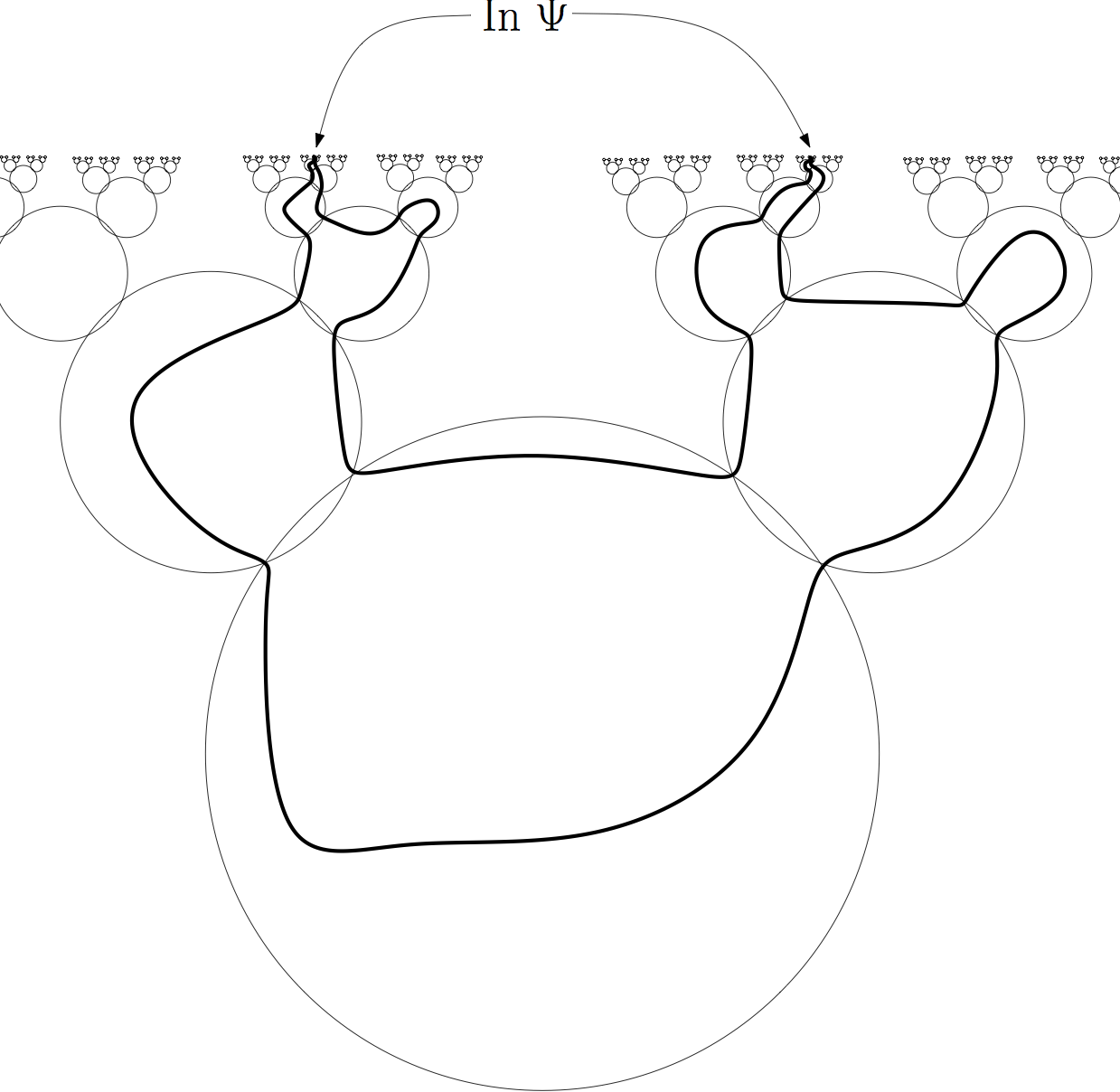Unfortunately, this construction does not always give a matroid. The reason why not is related to some tricky set-theoretical issues, so we will not discuss it here. But due to a very deep and beautiful set-theoretic result called Borel Determinacy, we know that this construction will give a matroid if the set $\Psi$ is topologically nice enough (if it is a Borel set) [BC15].

There are also certain infinite trees of matroids such that the above construction will give a matroid for any set $\Psi$ of ends. This has the immediate consequence that there are a lot of infinite matroids – as many as there could possibly be. A matroid is determined by a set of subsets of its ground set, so there could be no more than $2^{2^{\aleph_0}}$ matroids on a countable set. And in fact, there are that many non-isomorphic matroids on a countable set, because there are that many possibilities for the set $\Psi$.

This means that when an infinite matroid is constructed from a tree of matroids together with a set $\Psi$ of ends, most of the information is encoded in the set $\Psi$ – if the matroids in the tree are finite, then there are only $2^{\aleph_0}$ possibilities for the tree of matroids, but there are $2^{2^{\aleph_0}}$ possibilities for $\Psi$. This huge reservoir of extra information hidden at the ends means that countable matroids behave in many ways like uncountable graphs. For example, the constructions which show that infinite graphs in general are not well-quasi-ordered under the minor relation already work for countable matroids, even though they do not work for countable graphs [BC15].

The construction we are considering also plays a key role for the reconstruction of infinite matroids from their decompositions into 3-connected parts. It is known that any finite matroid is canonically expressible as a 2-sum of a finite tree of matroids, each of which is either 3-connected or else consists of a single circuit or cocircuit [CE80, S80]. This result extends directly to infinite matroids: any matroid can be canonically expressed as a 2-sum (in the above sense) of a tree of matroids, each of which is either 3-connected or else consists of a single circuit or cocircuit [ADP15, BC16]. As with the finite result, this implies that it often suffices to prove a result only for 3-connected matroids in order to be able to deduce it for all matroids.

The construction we have considered was based on the 2-sum, one of the simplest possible ways to stick matroids together. Next time, we shall see that similar ideas work to give infinitary versions of other more complicated ways of sticking matroids together, and the surprisingly close connections between these infinitary sums and the matroids naturally arising in infinite graphs.

[ADP15] E. Aigner-Horev, R. Diestel, L. Postle. The structure of 2-separations of infinite matroids, to appear in JCTB, available here.
[BC15] N. Bowler and J. Carmesin, Infinite Matroids and Determinacy of Games, submitted to LMS, available here.
[BC16] N. Bowler and J. Carmesin, The ubiquity of Psi-matroids, preprint, available here.
[CE80] W. H. Cunningham and J. Edmonds, A combinatorial decomposition theory, Canad. J. Math. 32 (1980), no. 3, 734–765.
[S80] P. D. Seymour, Decomposition of regular matroids, J. Combin. Theory Ser. B 28 (1980), no. 3, 305–359.